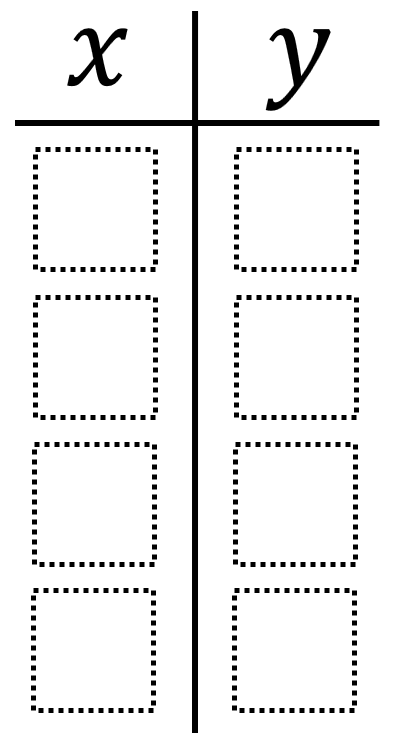# Linear Function from Table of Values

Directions: Using the digits 1 to 9 at most one time each, fill in the boxes to create a table of values that represent a linear function.### Hint

What has to happen for a table to show a linear relationship? What has to happen for a table to NOT show a linear relationship?

Here are two possible solutions. The first number is the x-value and the second number is the y-value.

Solution 1
1 2
3 4
5 6
7 8

Solution 2
1 5
2 6
3 7
4 8

Source: Robert Kaplinsky

## Square Root Expression 2

Directions: Using the digits 0 to 9 at most one time each, place a digit …

1.Can we swap x and y and create two more solutions?

2.x:y
1:3
2:7
3:7
4:4
7:1
5:7

3.Using digits 1 through 4 at most once each, there are 9 different ways to complete the tables to create a table of values ​​that represents a linear function.

4.x y
1 1
2 3
3 6
4 9

5.X Y
12:14
14:15
16:16